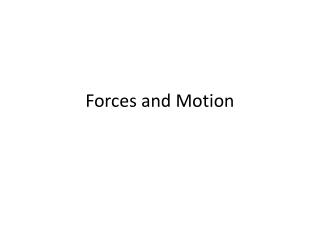Download PresentationForces and Motion# Forces and Motion - PowerPoint PPT Presentation

Download Presentation##### Forces and Motion

Download Policy: Content on the Website is provided to you AS IS for your information and personal use and may not be sold / licensed / shared on other websites without getting consent from its author. While downloading, if for some reason you are not able to download a presentation, the publisher may have deleted the file from their server.

- - - - - - - - - - - - - - - - - - - - - - - - - - - E N D - - - - - - - - - - - - - - - - - - - - - - - - - - -
##### Presentation Transcript

1. Forces and Motion

2. Gravity • Objects of different masses fall at the same rate. • Galileo work • Acceleration depends on force and mass

3. Change in velocity equation • Δ v = g X time • The change in velocity ( Δ ) is the difference between the final velocity and the starting velocity.

4. Formulas for Velocity of falling objects • t = Δv/g or • Δ V = g X t or • . g = Δv / t

5. 3 effects of Air resistance on a falling object • 1. Force of gravity= 9.8 m/s2 • 2. Force of air resistance= is the force pushing up on an apple • 3. Net force= Fg -Far

6. Falling objects • Leaf or Acorn

7. Free Fall • Facts about Free fall • A. No Air resistance • B. Only happens when you have gravity and no other force • C. Free fall can occur only when there is no air • D. Happens in space and in a vacuum • E. Orbiting objects are in free fall

8. Orbits • Need a forward motion • Need a gravity force • Both are needed to keep an object in orbit

9. Centripetal force • Is an unbalanced force that causes objects to move in a circular path • Usually this force is gravity

10. Projectile motion • Is the curved path an object follows when it is thrown or propelled near the surface of the earth. • It has two components a horizontal and a vertical motion which are independent of each other

11. Projectile Motion examples • 1. A frog leaping • 2. Water being sprayed by a sprinkler • 3. A swimmer diving into water • 4. Balls being juggled • 5. An arrow shot by an archer

12. Newton’s Laws of Motion • First Law of Motion = An object at rest remains at rest and an object in motion remains in motion at constant speed and in a straight line unless acted on by an unbalanced force.

13. Newton’s Laws of Motion • Newton’s second law of motion- The acceleration of an object depends on the mass of the object and the amount of force applied.

14. Newton’s Laws of Motion • Newton’s third law of motion= Whenever one object exerts a force on a second object the second object exerts an equal and opposite force on the first.

15. Friction • An unbalanced force affects Newton’s first law of motion. This unbalanced force is friction. • Because of friction the motions of objects changes

16. Inertia • Is the tendency of all objects to resist any change in motion. • Law of Inertia aka Newton’s first law of motion • Mass is a measure of Inertia

17. Newtons • Newton = N • N = kg * m /s2

18. Formulas for 2nd Law • F = m X a or • A = F/m or • M = F/a

19. 3rd law • Force pairs do not act on the same object • All forces act in pairs • The effect of a reaction can be difficult to see

20. Momentum • Is a quantity defined as the product of the mass and velocity of an object • Momentum is known as p

21. Formulas for P • P = m X v or • . m = p/v or • . v = p/m

22. Law of conservation of Momentum • States that any time objects collide the total amount of momentum stays the same • This law is true for any collision if no other forces act on the colliding objects. • This law applies whether the objects stick together of bounce off each other after they collide.